### 4.2. Topic-focus articulation and the semantic type of dependency relation (functor)

The semantic type of dependency relation (i.e. functor) can be helpful in annotating the contextual boundness of a modification. Modifications with certain functors are usually contextually bound, while modifications with other functors tend to be contextually non-bound.

There are the following tendencies in the relationship between topic-focus articulation and functors:

• modifications with the following functors tend to be contextually bound :

• the functor `PREC`.

All particles and paratactic connectives that signal connection to the preceding context are always non-contrastive contextually non-bound modifications (e.g.: tedy (=so), proto (=therefore), však (=however), tudíž (=hence), totiž (=thus) etc.), i.e. modifications with the functor `PREC` (see Section 7.4, "PREC"). Their primary function is to integrate the sentence into the context.

• the functor `ATT`.

Also attitudinal particles, i.e. modifications with the functor `ATT`, are usually non-contrastively contextually bound (see Section 7.1, "ATT").

Example:

Naštěstí.`ATT` [`tfa`=`t`] přišel [`tfa`=`f`] (=lit. Fortunately (he) came.) Fig. 10.14

• the functor `VOCAT`.

Modifications with the functor `VOCAT` are also usually non-contrastively contextually bound (see Section 1.3, "VOCAT"), because they refer to the addressee of the text, who is part of the situational context. Vocatives usually stand at the beginning of sentences, which is also the position in tectogrammatical trees at which nodes with the `VOCAT` functor are placed. We move them to the first position in the tectogrammatical tree even if in the surface word order they stand somewhere else.

Example:

Jirko.`VOCAT` [`tfa`=`t`] , podej [`tfa`=`f`] mi [`tfa`=`t`] pero [`tfa`=`f`] (=lit. George, pass me (the) pen.) Fig. 10.15

• modifications with the following functors tend to be contextually non-bound:

• functors for noun modifications (`RSTR`, `APP`, `MAT` or `ID`).

Most noun modifications, i.e. modifications with adnominal functors (see Section 10, "Specific adnominal functors"), especially with the functor `RSTR`, tend towards contextual non-boundness because their function is to specify their governing nouns. Nodes representing adnominal modifications expressed by adjectives, nouns, pronouns or numerals are therefore usually assigned the `tfa` value `f`, even though they are placed to the left from the noun in the surface word order. We assign the value `t` only when the particular attribute is repeated or is obvious from the preceding context.

Example:

Ve starém.`RSTR` [`tfa`=`f`] parku [`tfa`=`t`] stál [`tfa`=`f`/`t`] starý.`RSTR` [`tfa`=`t`] dům [`tfa`=`f`] (=lit. In (an) old park stood (an) old house.) Fig. 10.16

• functors for manner and its specific variants (`MANN` etc.).

Adverbs of manner that specify the event expressed by a verb, i.e. modifications with the functors for manner and its specific variants (see Section 6, "Functors for expressing manner and its specific variants"), especially with the functor `MANN`. Nodes representing these modifications are therefore usually assigned the `tfa` value `f` and are moved to the right after the verb (even though they stand to the left from it in the surface word order).

Example:

Rychle.`MANN` [`tfa`=`f`] přišel [`tfa`=`f`] (=lit. Quickly (he) came.) Fig. 10.17

• the functor `MOD`.

Also modal adverbials, i.e. modifications with the functor `MOD` (see Section 7.3, "MOD"), specifying the modality of the governing event, are contextually non-bound.

• the functor `DENOM`.

Nodes with the functor `DENOM` (see Section 1.2, "DENOM"), i.e. effective roots of nominative clauses (titles, lists etc.), are usually contextually non-bound. Nominative clauses are usually not related to context, they do not contain contextually bound expressions. In most cases, the whole nominative clause is contextually non-bound.

Example:

Škola.`DENOM` [`tfa`=`f`] šachových [`tfa`=`f`] koncovek [`tfa`=`f`] (=lit. School of_chess ending_games.) Fig. 10.18

• the functor `PAR`.

The effective roots of syntactically non-incorporated parentheses, i.e. nodes with the functor `PAR` (see Section 1.5, "PAR"), are also usually contextually non-bound. The underlying order of nodes with the functor `PAR`, however, corresponds to their surface order because they represent independent clauses not integrated into the structure of the sentence.

This is an exception from the basic guidelines for the ordering of nodes on the tectogrammatical level (see Section 3.1, "Basic guidelines for the ordering of nodes in a tectogrammatical tree").

The behaviour of the so-called rhematizers (`functor`=`RHEM`) is very specific and we describe it in Section 6, "Rhematizers".

Figure 10.14. Topic-focus articulation and the semantic type of dependency relation (functor)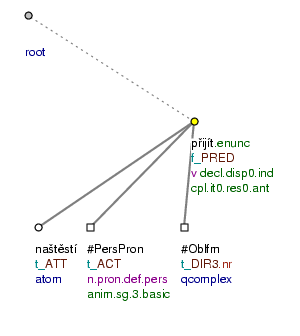Naštěstí přišel. (=lit. Fortunately (he) came.)

Figure 10.15. Topic-focus articulation and the semantic type of dependency relation (functor)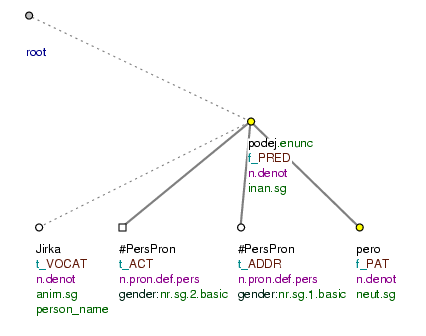Jirko, podej mi pero. (=lit. George, pass me (the) pen.)

Figure 10.16. Topic-focus articulation and the semantic type of dependency relation (functor)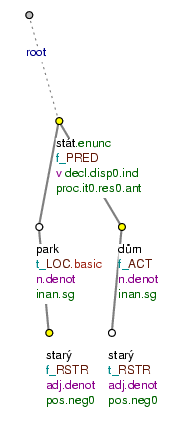Ve starém parku stál starý dům. (=lit. In (an) old park stood (an) old house.)

Figure 10.17. Topic-focus articulation and the semantic type of dependency relation (functor)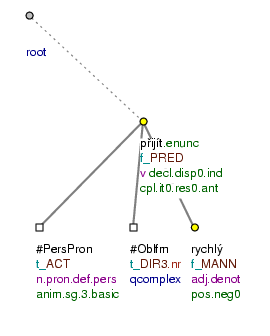Rychle přišel. (=lit. Quickly (he) came.)

Figure 10.18. Topic-focus articulation and the semantic type of dependency relation (functor)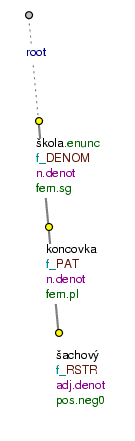Škola šachových koncovek (=lit. School of_chess ending_games.)